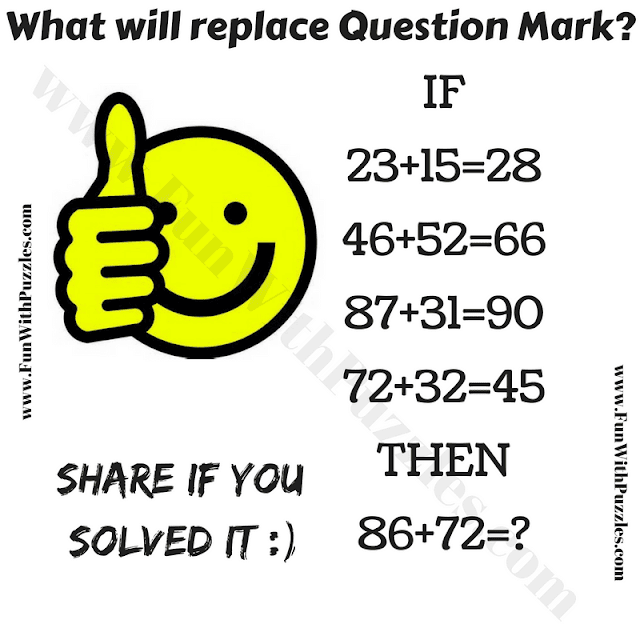This Mind Twisting Maths Question is for school-going teens. In this brain-twisting Maths question, there are some Mathematical equations given in the puzzle picture. However these equations look incorrect Mathematically, but there is a certain pattern that makes these equations correct. This pattern is applied to all the given equations. Your challenge is to find this hidden pattern and then find the value of the missing number which will replace the question mark?Can you solve this mind-twisting Maths Question?

The answer to this "Mind Twisting Maths Question", can be viewed by clicking on the button. Please do give your best try before looking at the answer.